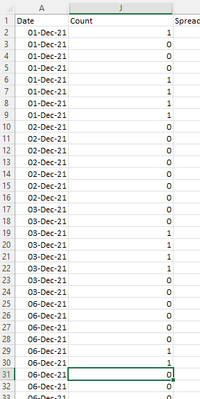cancel
Showing results for
Did you mean:Frequent Visitor

## Return value associated with latest date

Hi All

I am still relatively new in my DAX journey and looking for some assitance. I have a snippet of a table below and I have tried to create a calculated measure that

1. sum of the count  for latest date in table so below this would be 2 (6th Dec)

2. sum of the count  for second date in table so below this would be 4 (3rd Dec)I managed to get the required figure for point 1 but want this to be independent of other variables so always remains constant

Latest CMI Count = CALCULATE('Table'[Count Total],filter(ALL('Table'[Date]),'Table'[Date]=max('Table'[Date])))

1 ACCEPTED SOLUTIONSuper User

This will ignore any filters from anywhere

``````Prev CMI Count =
VAR MaxDate =
CALCULATE ( MAX ( 'Table'[Date] ), REMOVEFILTERS () )
VAR PrevDate =
CALCULATE ( MAX ( 'Table'[Date] ), 'Table'[Date] < MaxDate, REMOVEFILTERS () )
VAR Result =
CALCULATE ( [Count total], REMOVEFILTERS (), 'Table'[Date] = PrevDate )
RETURN
Result
``````
4 REPLIES 4Super User

Try

``````Prev CMI Count =
VAR MaxDate =
MAX ( 'Table'[Date] )
VAR PrevDate =
CALCULATE ( MAX ( 'Table'[Date] ), 'Table'[Date] < MaxDate )
VAR Result =
CALCULATE ( [Count total], REMOVEFILTERS ( 'Table' ), 'Table'[Date] = PrevDate )
RETURN
Result
``````Frequent Visitor

Thanks @johnt75

Have tried this to get the max and the second max and have obtained the correct counts but these change if I have any slicers on my dashboard. Can the DAX formula be modified so the result is constant?Super User

This will ignore any filters from anywhere

``````Prev CMI Count =
VAR MaxDate =
CALCULATE ( MAX ( 'Table'[Date] ), REMOVEFILTERS () )
VAR PrevDate =
CALCULATE ( MAX ( 'Table'[Date] ), 'Table'[Date] < MaxDate, REMOVEFILTERS () )
VAR Result =
CALCULATE ( [Count total], REMOVEFILTERS (), 'Table'[Date] = PrevDate )
RETURN
Result
``````Frequent Visitor

Excellent @JohnT this has now worked and above is understood. Thanks again!